Download Presentation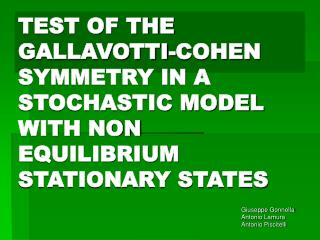TEST OF THE GALLAVOTTI-COHEN SYMMETRY IN A STOCHASTIC MODEL WITH NON EQUILIBRIUM STATIONARY STATES

# TEST OF THE GALLAVOTTI-COHEN SYMMETRY IN A STOCHASTIC MODEL WITH NON EQUILIBRIUM STATIONARY STATES - PowerPoint PPT Presentation

TEST OF THE GALLAVOTTI-COHEN SYMMETRY IN A STOCHASTIC MODEL WITH NON EQUILIBRIUM STATIONARY STATES. Giuseppe Gonnella Antonio Lamura Antonio Piscitelli. Equilibrium stationary states. Gibbs-Boltzmann distribution. Non equilibrium stationary states (N.E.S.S.). -Thermal gradientI am the owner, or an agent authorized to act on behalf of the owner, of the copyrighted work described.
Download Presentation## TEST OF THE GALLAVOTTI-COHEN SYMMETRY IN A STOCHASTIC MODEL WITH NON EQUILIBRIUM STATIONARY STATES

An Image/Link below is provided (as is) to download presentation

Download Policy: Content on the Website is provided to you AS IS for your information and personal use and may not be sold / licensed / shared on other websites without getting consent from its author.While downloading, if for some reason you are not able to download a presentation, the publisher may have deleted the file from their server.

- - - - - - - - - - - - - - - - - - - - - - - - - - E N D - - - - - - - - - - - - - - - - - - - - - - - - - -
Presentation Transcript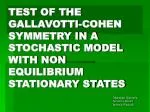### TEST OF THE GALLAVOTTI-COHEN SYMMETRY IN A STOCHASTIC MODEL WITH NON EQUILIBRIUM STATIONARY STATES

Giuseppe Gonnella

Antonio Lamura

Antonio Piscitelli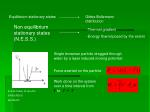Equilibrium stationary states

Gibbs-Boltzmann distribution

Non equilibrium stationary states (N.E.S.S.)

-Energy flow imposed by the extern

Single brownian particle dragged through water by a laser induced moving potential

Force exerted on the particle

Work done on the system over a time interval from

to

E.G.D.Cohen, R.van Zon

PHYS.REV.E

69,056121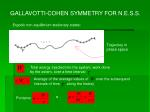GALLAVOTTI-COHEN SYMMETRY FOR N.E.S.S.

Ergodic non equilibrium stationary states:

Trajectory in phase space

Total energy injected into the system, work done by the extern, over a time interval

Average of the average values of

over the subsequent

intervals of time

along the history

e

PoniamoProbability distribution function that the ratio

assumes the value

in the time interval

The theorem suggests that:

in a non equilibrium stationary state the probability distribution function

satisfies that

where

is called Symmetry Function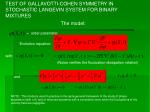TEST OF GALLAVOTTI-COHEN SYMMETRY IN STOCHASTIC LANGEVIN SYSTEM FOR BINARY MIXTURES

The model:

= order parameter

Evolution equation:

with:

(Noise verifies the fluctuation-dissipation relation)

and

NOTE: This model is used in practise in the quite general framework of the study of phase separation (with r<0) and of mixtures dynamics with a convective term, when the fluctuations of the velocity field are negligible.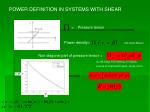POWER DEFINITION IN SYSTEMS WITH SHEAR

= Pressure tensor

Power density=

(De Groot-Mazur)

Non diagonal part of pressure tensor =

(A.J.M.Yang, P.D.Fleming, J.H.Gibbs,

Journal of Chemical Physics, vol.64, No.9)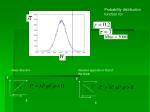Probability distribution function for

shear direction

direction opposite to that of the shear

y

y

x

x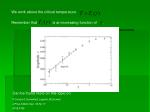We work above the critical temperature

Remember that

is an increasing function of .

Can be found more on this topic on

F.Corberi,G.Gonnella,E.Lippiello,M.Zannetti,

J.Phys.A:Math.Gen. 36 No 17

4729-4755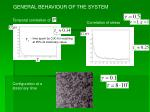GENERAL BEHAVIOUR OF THE SYSTEM

Temporal correlation of

Correlation of stress

time spent by C(X) for reaching at 95% its stationary value.

Configuration at a stationary time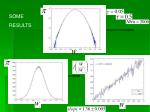SOME

RESULTSCONCLUSIONS

It has been measured the correlation time (of and ) and it has been verified that the characteristics and the behaviour of the system are typical of a stationary state above the critical temperature.

From the simulations performed until now

the limit slope (1 for GC symmetry) seems to vary with

and in particular seems to increase with

NEXT STEPS

To state with more precision the above result for understanding better the trend of the limit slope with

To approach the problem analytically